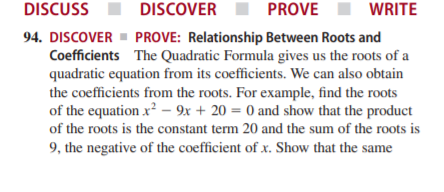# DISCUSS DISCOVER PROVE WRITE 94. DISCOVER ▪ PROVE: Relationship Between Roots and Coefficients The Quadratic Formula gives us the roots of a quadratic equation from its coefficients. We can also obtain the coefficients from the roots. For example, find the roots of the equation x? – 9x + 20 = 0 and show that the product of the roots is the constant term 20 and the sum of the roots is 9, the negative of the coefficient of x. Show that the same

Question

DISCOVER ■ Prove: Relationship Between Roots and
Coefficients The Quadratic Formula gives us the roots of a
quadratic equation from its coefficients. We can also obtain
the coefficients from the roots. For example, find the roots
of the equation x2  9x  20  0 and show that the product
of the roots is the constant term 20 and the sum of the roots is
9, the negative of the coefficient of x. Show that the samehelp_outlineImage TranscriptioncloseDISCUSS DISCOVER PROVE WRITE 94. DISCOVER ▪ PROVE: Relationship Between Roots and Coefficients The Quadratic Formula gives us the roots of a quadratic equation from its coefficients. We can also obtain the coefficients from the roots. For example, find the roots of the equation x? – 9x + 20 = 0 and show that the product of the roots is the constant term 20 and the sum of the roots is 9, the negative of the coefficient of x. Show that the same fullscreen

### Want to see this answer and more?

Experts are waiting 24/7 to provide step-by-step solutions in as fast as 30 minutes!*

*Response times may vary by subject and question complexity. Median response time is 34 minutes for paid subscribers and may be longer for promotional offers.
Tagged in
Math
Algebra

### Equations and In-equations